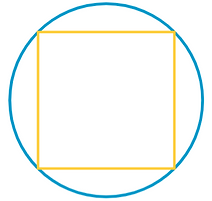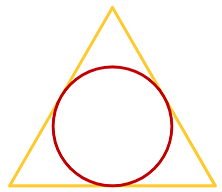Suanfa Tong Zong

In 1592, Chinese mathematician Cheng Dawei published Suanfa Tong Zongtranslated in English as either General Source of Computational Methods or Systematic Treatise on Mathematics. The text became a reference book for both professional and amateur mathematicians, with a great influence on the development of Japanese mathematics as well.

Cheng Dawei

A portrait of Cheng DaweiThe problems below appeared in chapters 3, 7, and 12,  respectively. In the first six we are asked to show that each given relationship between the area of a circle and that of a regular polygon is true.

Suanfa Tong Zong

Sources: Sacred mathematics: Japanese Temple Geometry by Fukagawa Hidetoshi and Tony Rothman (2008); A History of Chinese Mathematics by Jean-Claude Martzloff (2006).

Triangle in CircleThe area of the equilateral triangle is about seven sixteenths the area of the circle.

Square in CircleThe area of the square is about two thirds the area of the circle.

Hexagon in CircleThe area of the regular hexagon is about six sevenths the area of the circle.

Circle in TriangleThe area of the circle is about four sevenths the area of the equilateral triangle.

Circle in SquareThe area of the circle is about three fourths the area of the square.

Circle in HexagonThe area of the circle is about six sevenths the area of the regular hexagon."How Deep is the Pond?" problem as illustrated in Suanfa Tong Zong

How Deep is the Pond?Two reeds of equal length project 3 syaku above the pond's surface. One reed is pulled 9 syaku towards the shore such that it is now just touching the surface. How deep is the pond?

An Equilateral TriangleAn equilateral triangle of side 20 is divided into three equal parts. What are the area and the side lengths of each quadrilateral?

Right Triangle and CircleWhat is the radius of the circle?

Right Triangle and SquareWhat is the side of the square?